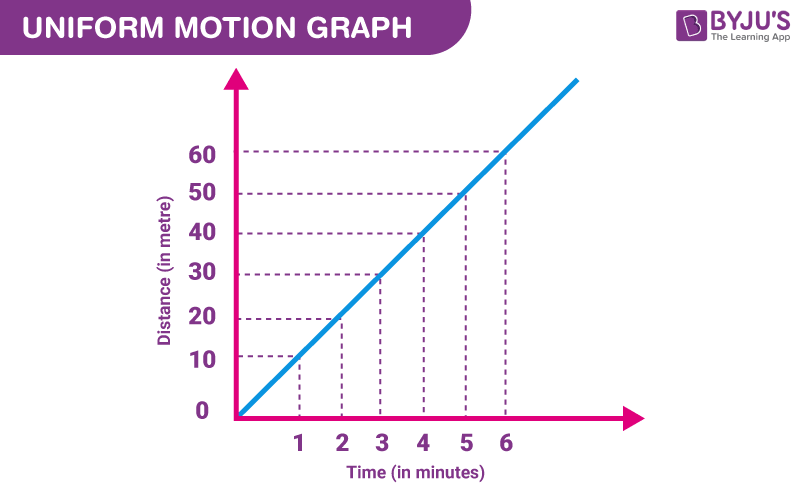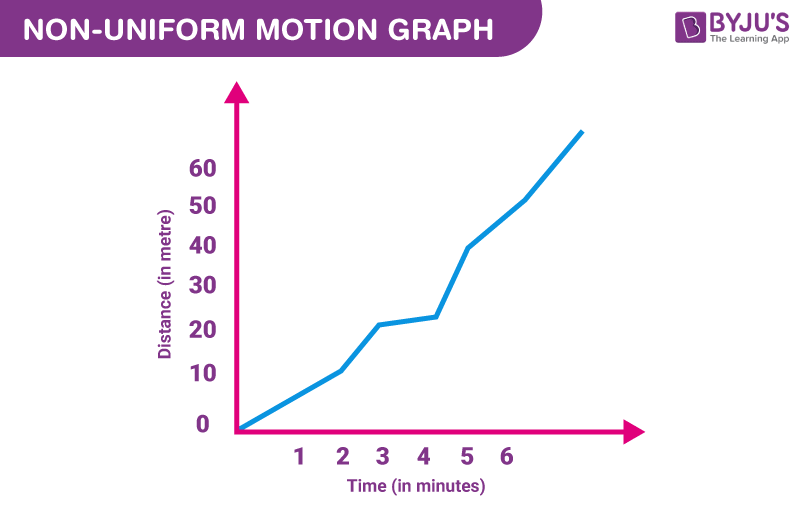# Uniform Motion and Non Uniform Motion

## Uniform Motion:

Definition: This type of motion is defined as the motion of an object in which the object travels in a straight line, and its velocity remains constant along that line as it covers equal distances in equal intervals of time, irrespective of the duration of the time.If a body is involved in rectilinear motion and the motion is consistent, then the acceleration of the body must be zero.

### Example of Uniform Motion:

1. If the speed of a car is 10 m/s, it means that the car covers 10 meters in one second. The speed is constant every second.
2. Movement of blades of a ceiling fan.

## Non Uniform Motion:

Definition: This type of motion is defined as the motion of an object in which the object travels with varied speed and it does not cover the same distance in equal time intervals, irrespective of the time interval duration.If a body is involved in rectilinear motion, and if the motion is not consistent, then the acceleration of the body must be non-zero.

## The below video provides the Top 10 NTSE Important Questions on Motion Class 9Test your Knowledge of Uniform Motion and Non-Uniform Motion!

### Example of Non Uniform Motion:

1. If a car covers 10 meters in the first two seconds and 15 meters in the next two seconds.
2. The motion of a train.

Now, people usually get confused between uniform motion and uniform acceleration. In the later phenomena, the object has a constant acceleration in rectilinear motion, which means the object has a different speed every second, which clearly defines that motion is changing.

Following is the table explaining concepts related to motion

### Comparison Table between These Two Types of Motions

Comparison Parameters

Uniform Motion

Non Uniform Motion

Average Speed

The motion is similar to the actual speed of the object.

The motion is different from the actual speed of the object.

Rectilinear Motion

It has zero acceleration.

It has non-zero acceleration.

Graph

Distance-time graph shows a straight line

Distance-time graph shows a curved line

Distance

Covers equal distances in the equal time interval.

Covers unequal distances in the equal time interval.### Uniform Circular Motion:

This type of motion is seen if an object is travelling at a constant speed around a fixed axis or centre point, the object travels around a curved path, and it maintains a constant radial distance from the centre point at any given time, and it moves in a tangent to the curved path.

### Non Uniform Circular Motion:

This type of motion is inconsistent because if an object is travelling at a variable angular speed around a fixed axis or centre point, then the motion of that object is said to be inconsistent because the object covers a curved path and it will have some variable radial acceleration due to which its velocity would change every second.

## Watch the video and solve important questions in the chapter Motion Class 9Stay tuned to BYJU’S and Fall in Love with Learning!

Test your Knowledge on Uniform Motion And Non-Uniform Motion

1. Thanks

2. Thankyou very much byjus

3. It is very useful

4. Best

5. can you please help me to show acceleration of a body is zero if it moves in uniform motion. Numerically with its formula

• Yes, you are right. The acceleration of a body in uniform motion is zero.

As an example, let us consider a body moving in uniform motion with a velocity of 5 m/s from point A to B. The total time taken to complete this distance is 3 seconds. What is the acceleration of the body?

The acceleration of the body is calculated using the following formula:
a = (v-u)/t
here, v is the final velocity and u is the initial velocity
Substituting the values in the equation, we get
a = (5-5)/3 = 0
As the body is in uniform motion, there is no change in velocity as a result the initial and final velocity is the same.

• Thanks

6. Is it necessary for the angle formed by the line on the x-axis and on the y-axis of uniform motion in a distance-time graph to be 45degree?

7. Thank you for ur great information!!

8. Thankyou so much. It helped me a lot in my project report

9. What is speed ?

• Speed is defined as the distance divided by time.

10. Thank you very much

11. Does a body always have to travel in a straight line for uniform motion?

• Yes, in a uniform motion the body travels in a straight line. Uniform motion is a type of motion in which a body has a constant speed and unvarying direction. This implies that the direction is fixed, in other words a “straight line.”